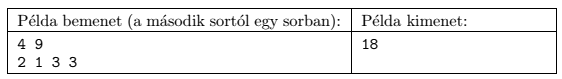Mathematical and Physical Journal
for High Schools
Issued by the MATFUND Foundation
 Already signed up? New to KöMaL?

#Problem S. 96. (February 2015)

S. 96. A beam of length $\displaystyle D$ ($\displaystyle 1\le D\le 10^{15}$) should be cut into $\displaystyle N$ pieces ($\displaystyle 1\le N \le 500\;000$). The length of the $\displaystyle i$th piece should be $\displaystyle r_i$ ($\displaystyle 1\le r_i\le 10^{15}$), and the sum of these $\displaystyle r_i$ numbers is $\displaystyle D$. The cost of cutting any beam of length $\displaystyle L$ into two arbitrary pieces is $\displaystyle L$ units of money. We always cut one piece into two smaller pieces at a time. Your task is to cut the original beam of length $\displaystyle D$ into smaller pieces with length $\displaystyle r_i$ so that the total cost is minimal.

Your program reads the values of $\displaystyle N$ and $\displaystyle D$ from the first line of the standard input, then the $\displaystyle r_i$ integers from the following $\displaystyle N$ lines. The first line of the standard output should contain the minimum sum necessary to complete the cutting process.

In the Példa bemenet'' sample input the $\displaystyle r_i$ integers are listed in one line, and the minimum cost is given in the Példa kimenet'' sample output.Scoring and bounds. You can obtain 1 point for a brief and proper documentation clearly describing your solution. Nine further points can be obtained provided that your program solves any arbitrary valid input within 1 second of running time.

The source code (s96.pas, s96.cpp, ...) without the .exe or any other auxiliary files generated by the compiler but with a short documentation (s96.txt, s96.pdf, ...), also describing which developer environment to use for compiling the source, should be submitted in a compressed file s96.zip.

(10 pont)

Deadline expired on March 10, 2015.

### Statistics:

 12 students sent a solution. 10 points: Csenger Géza, Juhász 326 Dániel, Weisz Ambrus, Zalavári Márton, Zarándy Álmos. 4 points: 1 student. 3 points: 3 students. 2 points: 1 student. 1 point: 1 student. 0 point: 1 student.

Problems in Information Technology of KöMaL, February 2015